The perfect place for easy learning...

×

# Python Data Types

The Python programming language provides a variety of built-in data types. The following are the most commonly used built-in data types in Python.

• None
• Numeric
• String
• List
• Tuple
• Set
• Range
• Dictionary

Let's look at each built-in data type in Python.

## 'None' data type in Python

In many programming languages, we use the value null to represent nothing. 'None' is Python's equivalent of NULL value in other programming languages. In Python, 'None' is the object which represents nothing. When we want a value to hold nothing, we do assign it with value 'None'.

# Example - Python code to illustrate 'None' data type

``````
roll_number = None
print(type(roll_number))
```
```

When we run the above code, it produces the output as follows.## 'Numeric' data type in Python

The Python programming language provides four numeric data types. They are as follows.

• int - All the numbers without fraction part (Example - 10). For int, there is no upper limit.
• float - All the numbers with a fraction part (Example - 10.5). It’s accurate up to 15 decimal places
• complex - All the numbers with real and imaginary parts (Example - 5 + 10j).
• bool - boolean values True and False.

# Example - Python code to illustrate 'Numeric' data type

``````
a = 10
print(f"the value {a} is of type {type(a)}")
a = 10.5
print(f"the value {a} is of type {type(a)}")
a = 100 + 5j
print(f"the value {a} is of type {type(a)}")
a = False
print(f"the value {a} is of type {type(a)}")
```
```

When we run the above code, it produces the output as follows.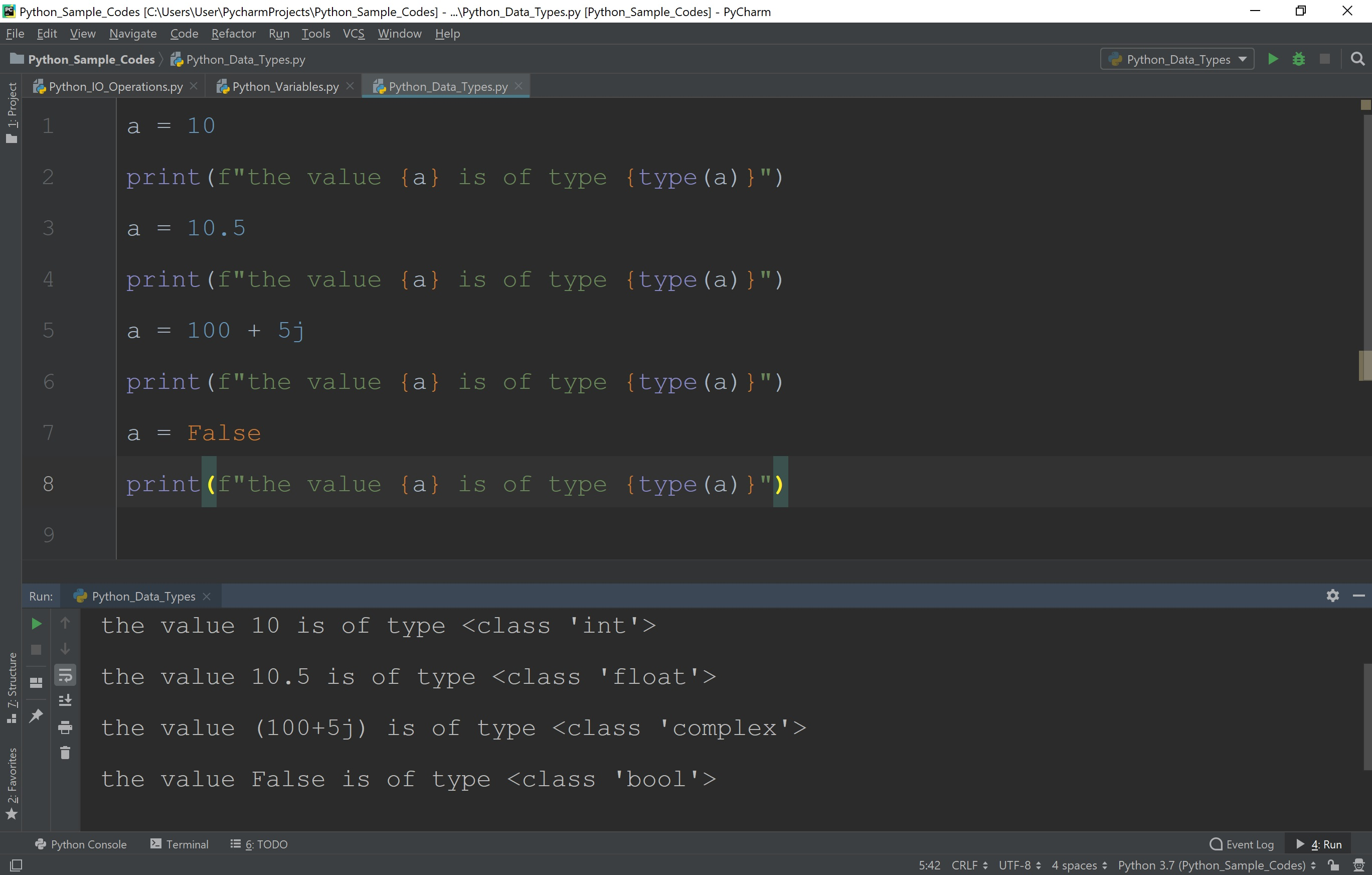## 'String' data type in Python

A string is a sequence of character data which are confined in either a single quote or double quote. The string data type in Python is called as 'str'. In Python, a string can contain as many characters as you wish. The only limit is the system's memory. In Python, the string may also be an empty string.

# Example - Python code to illustrate 'String' data type

``````
wish = 'Good Morning'
print(type(wish))
```
```

When we run the above code, it produces the output as follows.## Tips!

• When a string contains a single quotation as a character in it, then enclose it with double quotes.
(Example - "It's cool")
• When a string contains double quotation as a character in it, then enclose it with single quotes.
(Example - 'Today is so "hot" outside')
• When a string contains both double quotation and a single quotation as characters in it, then enclose it with triple quotes.
(Example - '''It's so "hot" outside''')

Let's look at the following Python code to illustrate quotations in a string value.

# Example - Python code to illustrate quotations in 'String'

``````
report = "It's so cool"
print(report)
report = 'Today is so "Hot" outside'
print(report)
report = '''It's so "Hot" outside'''
print(report)
```
```

When we run the above code, it produces the output as follows.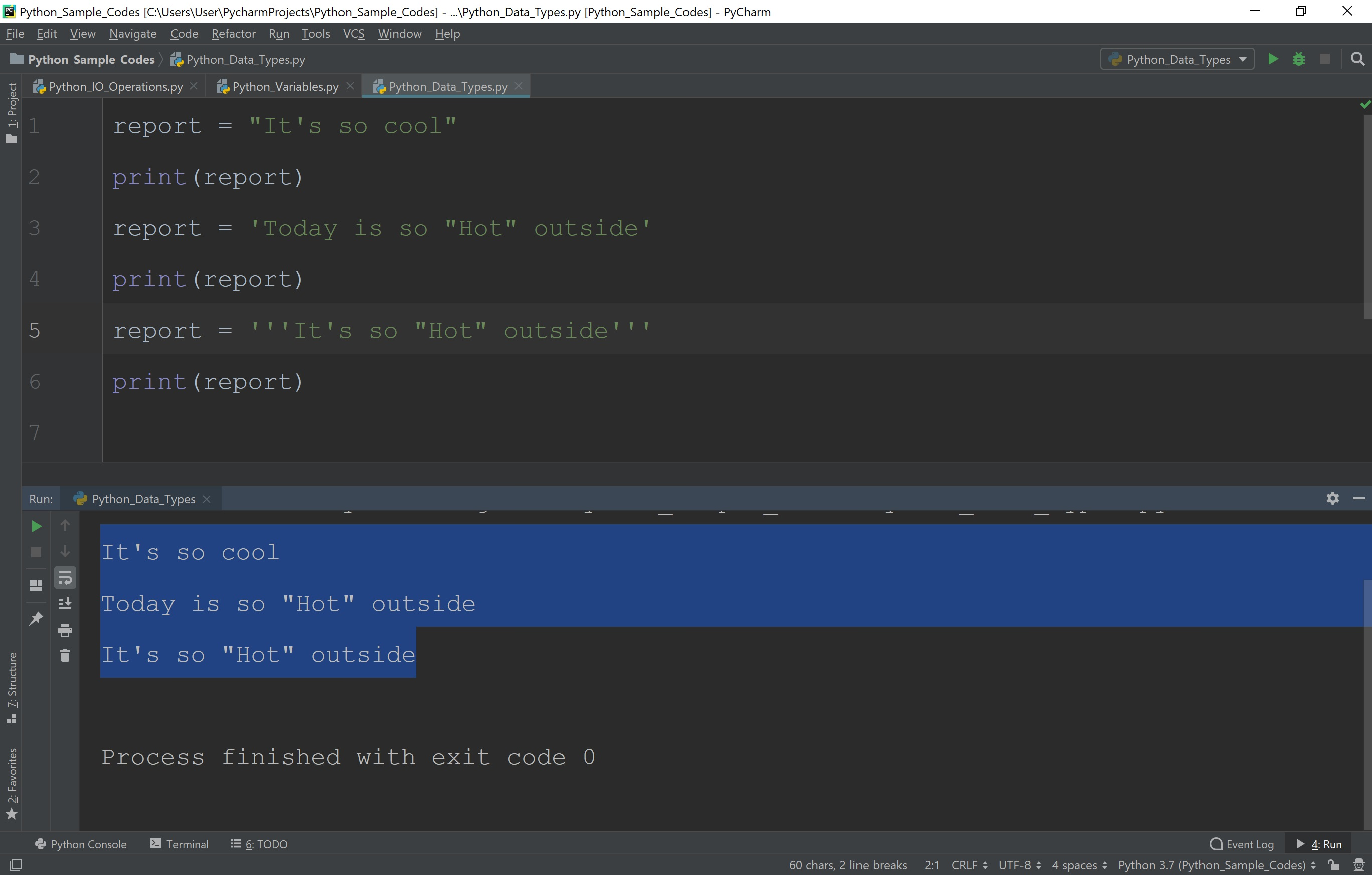## Escape Sequences in Python Strings

The escape sequences are the special characters in string data. All the escape sequences are prefixed with a backslash (\) character. A backslash (\) character in a string intimates that one or more characters that follow it should be interpreted with their special meaning only. The following table presents all escape sequences that are allowed in Python.

S.No. Escape Sequence Special Meaning
1 \' single quote (') character
2 \" Double quote (") character
3 \\ backslash (\) character
4 \a ASCII Bell character
5 \b ASCII Backspace character
6 \n ASCII Newline character
7 \t ASCII Horizontal Tab character
8 \u Unicode character with 16-bit hex value
9 \U Unicode character with 32-bit hex value
10 \v ASCII Vertical Tab character
11 \o Character with octal value
12 \x Character with hex value
13 \newline Backslash and newline ignored

## 'List' data type in Python

The list is the most commonly used data type available in Python. In Python, the list data type values are enclosed in square brackets ([ ]) and each value is separated with comma symbol. In Python, the list data type may contain different data type values.

# Example - Python code to illustrate 'List' in Python

``````
roll_list = [1,2,3,4,5]
print(roll_list)
student = [1,'Rama',"3rd Year",86.5]
print(student)
print(type(student))
```
```

When we run the above code, it produces the output as follows.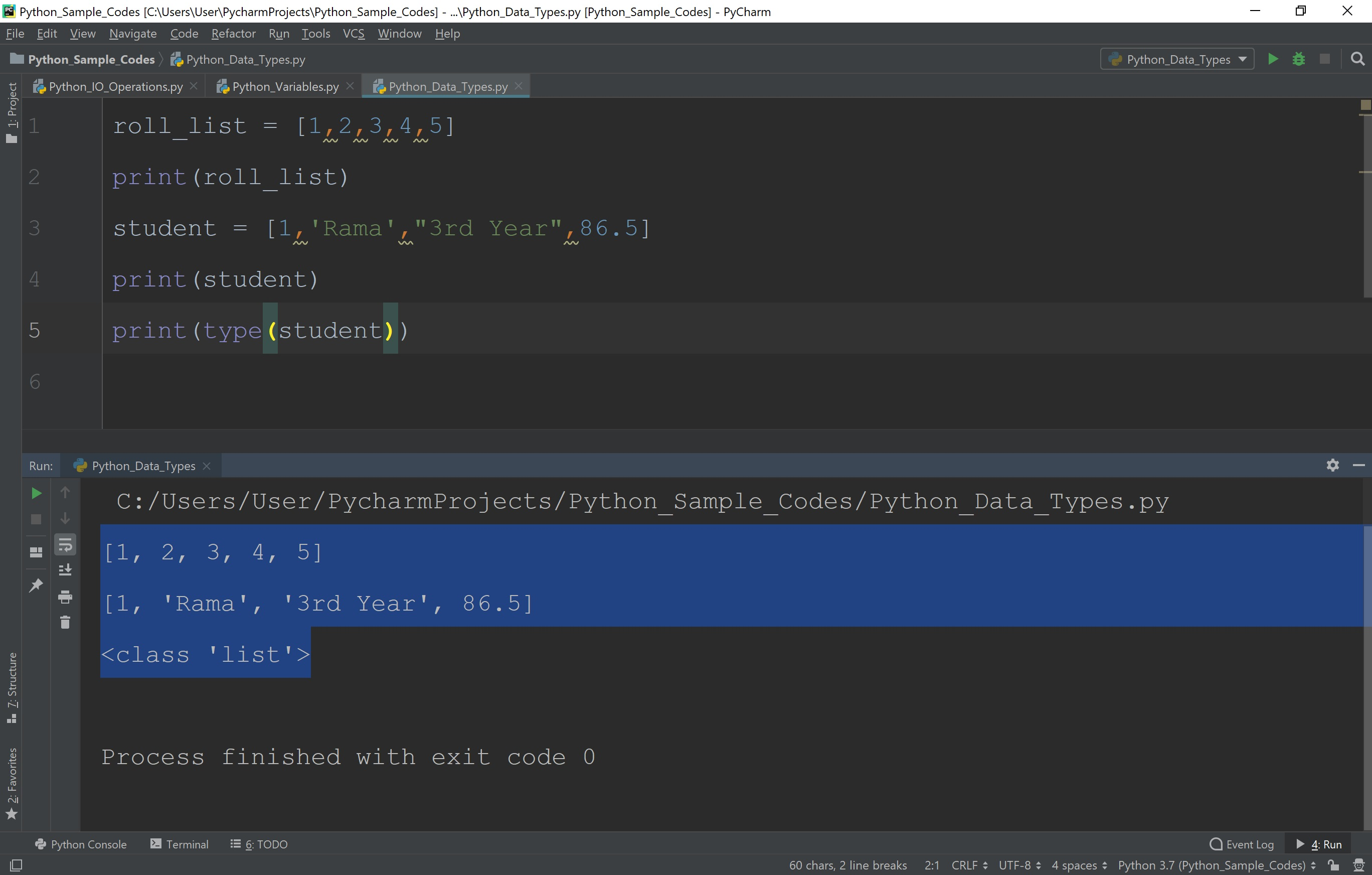## 'Tuple' data type in Python

The tuple is similar to list in Python. In Python, the tuple data type is immutable. That means the tuples cannot be modified, unlike lists. In Python, the tuples may contain different data type values. The tuples are enclosed in parentheses. Let's look at the code to illustrate tuples in Python.

# Example - Python code to illustrate 'Tuple' in Python

``````
roll_list = (1,2,3,4,5)
print(roll_list)
student = (1,'Rama',"3rd Year",86.5)
print(student)
print(type(student))
```
```

When we run the above code, it produces the output as follows.## 'Set' data type in Python

In Python, a set is a collection of an unordered and unindexed data elements of different data types. In Python, the set data type elements are immutable (duplicates are not allowed). However, set itself is mutable. Set is created with curly brackets. Let's look at the code to illustrate set in Python.

# Example 1 - Python code to illustrate 'set' in Python

``````
student = {1,'Rama',"3rd Year",86.5}
print(student)
print(type(student))
```
```

When we run the above code, it produces the output as follows.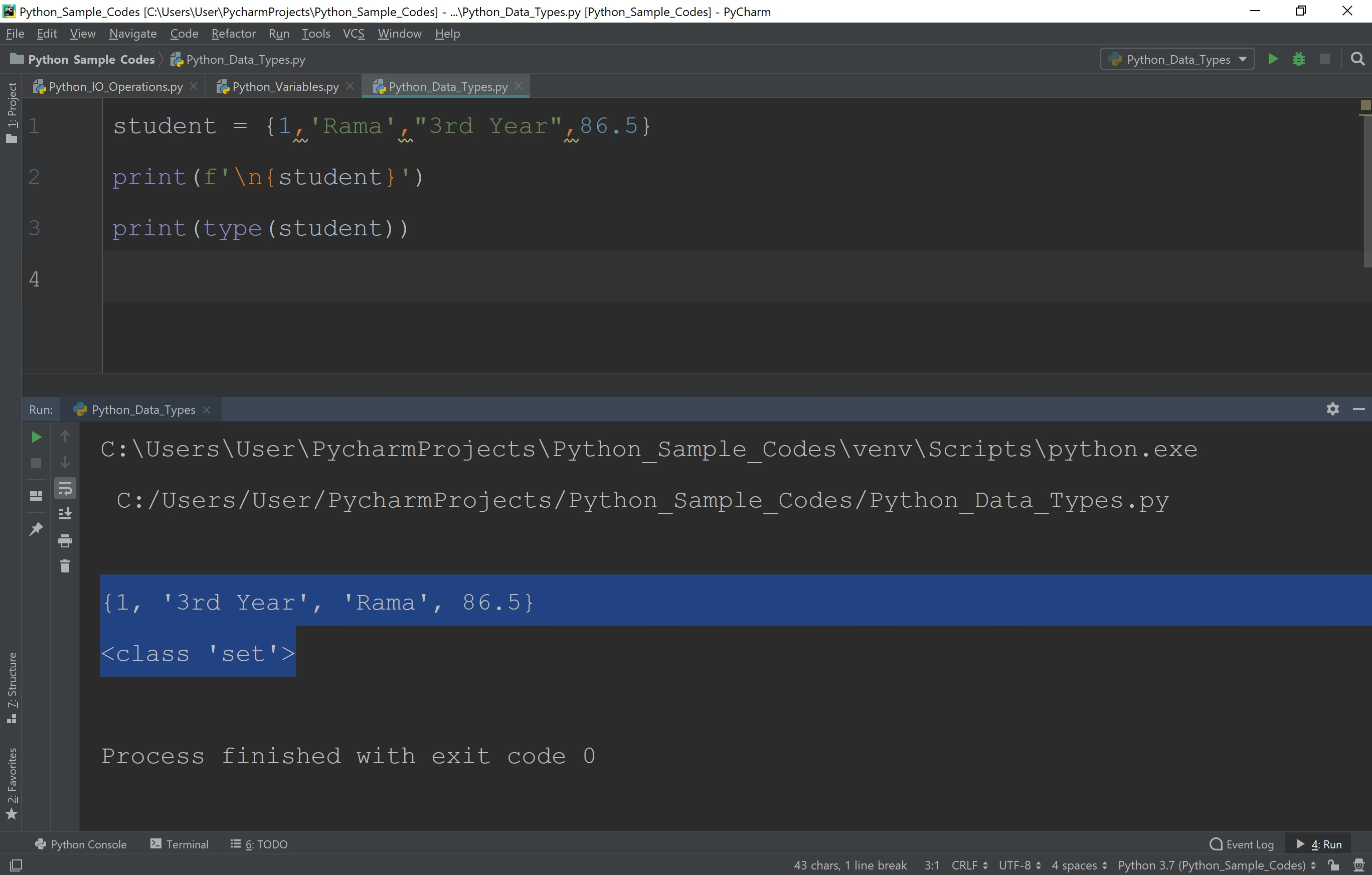We can also use the constructor 'set( )' to create a set in python. Let's look at the code to illustrate set using a constructor in Python.

# Example 1 - Python code to illustrate 'set' in Python

``````
student_1 = set((1,'Rama',"3rd Year",86.5))
student_2 = set([2,'Seetha',"2nd Year",89.5])
print(f'\n {student_1}\n {student_2}')
print(type(student_1), type(student_2))
```
```

When we run the above code, it produces the output as follows.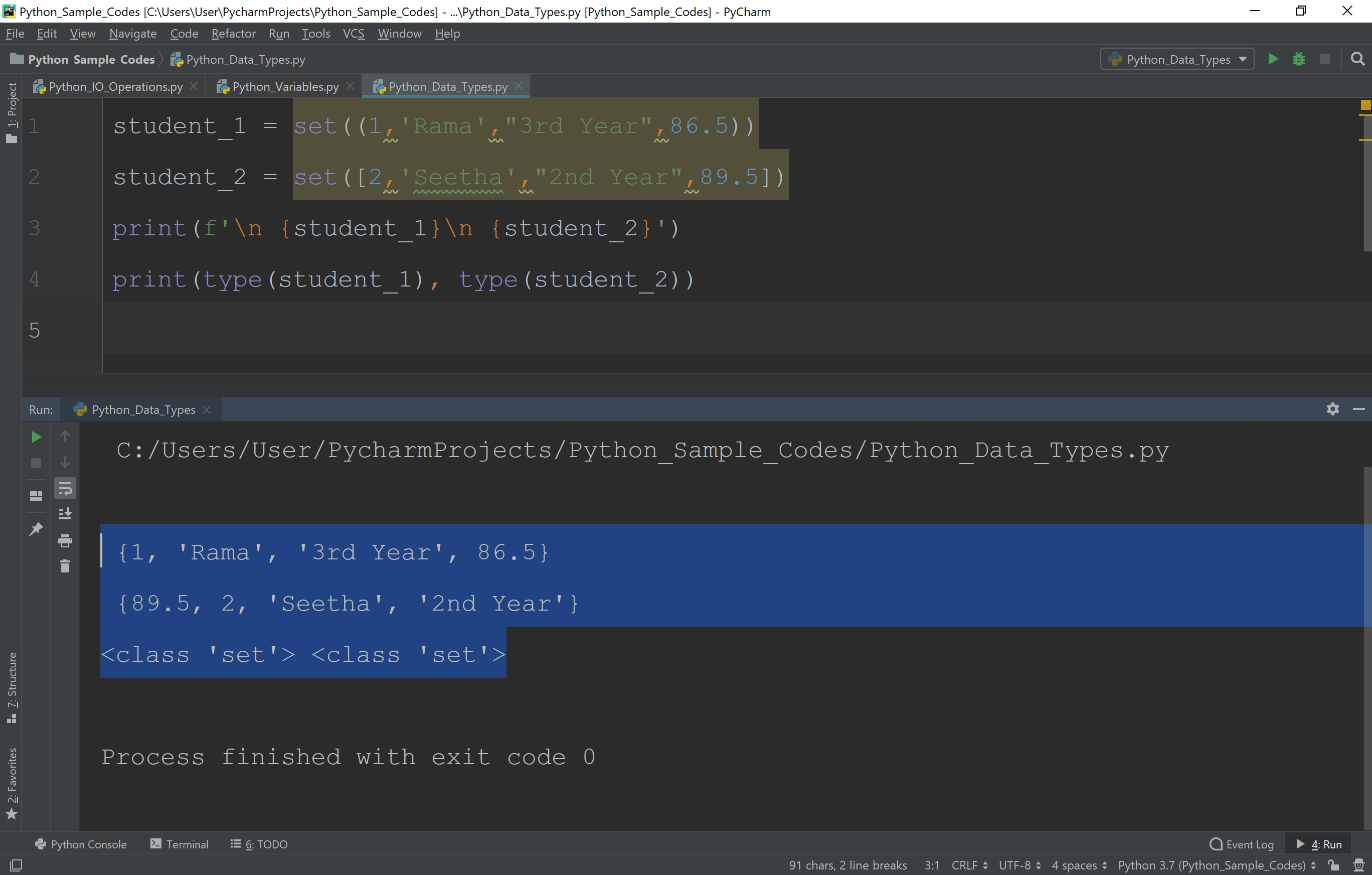## 'Range' data type in Python

In Python, range( ) is a built-in function used to create a list of integers with a specified range of values. The general syntax of range( ) function is as follows.

### Syntax

variable_name = range(start, end, difference)

Let's look at the following example python code to illustrate range( ) function in Python.

# Example 1 - Python code to illustrate 'range( )' in Python

``````
a = range(10,20,1)
print(type(a))
print(list(a))
```
```

In the above example Python code the variable a is stored with a list [10,11,12,13,14,15,16,17,18,19]. The range( ) function takes three arguments. The first argument indicates the starting value, the second argument indicates ending value, and the third argument indicates the difference between each element in the list.

When we run the above code, it produces the output as follows.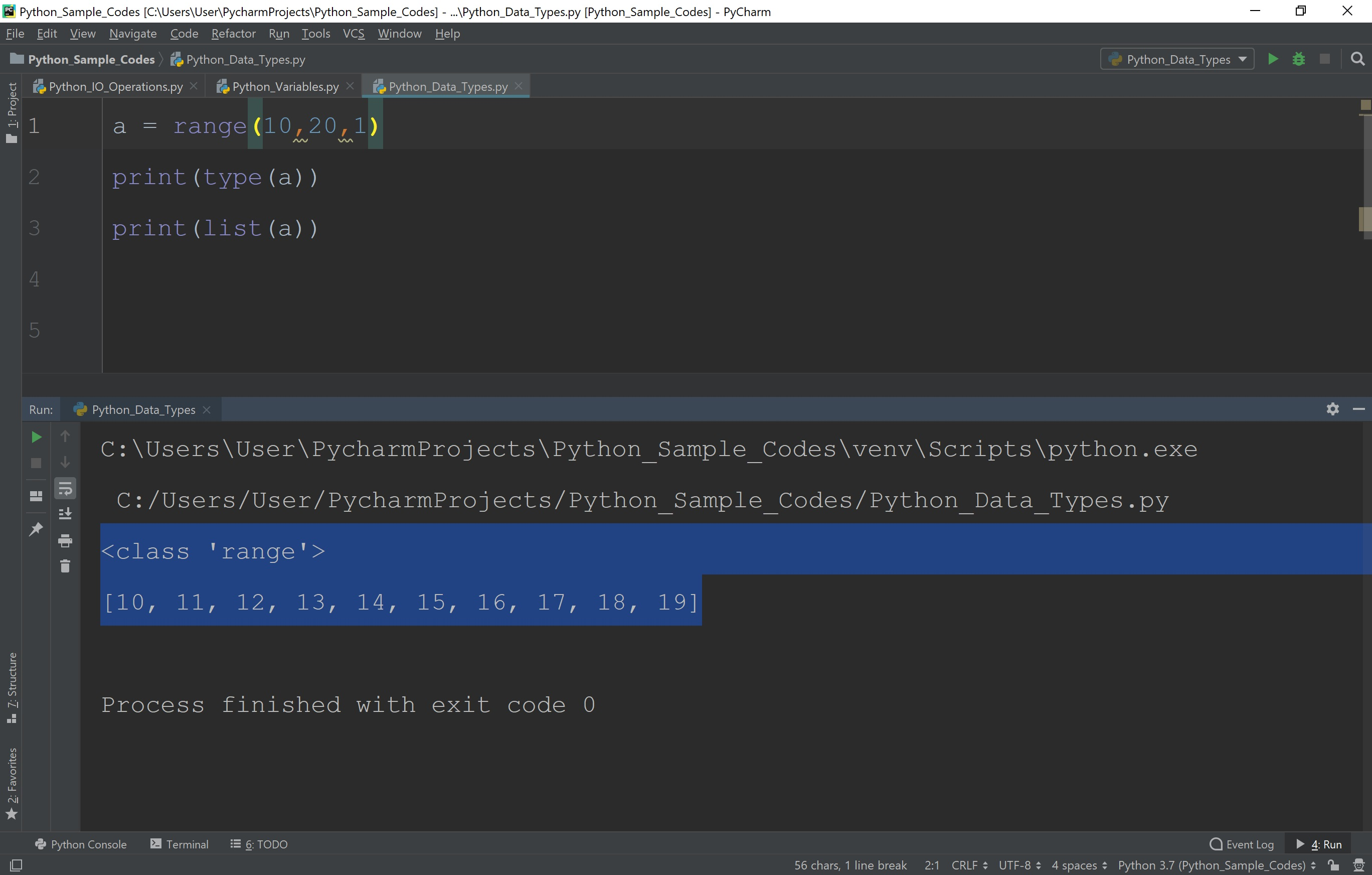• In Python, the default starting value of range( ) function is 0.
• In Python, the default difference between the values of range( ) function is 1.
• In Python, the ending value is not included in the list. That means, the range( ) function returns a list starting with starting value and ending with End-1 but end value is not included.

## 'Dictionary' data type in Python

In Python, a dictionary is similar to list, but dictionary stores each element as a pair of Key and Value. In list, every element is accessed using index value, whereas in the dictionary we use, a unique key to access a value. In a dictionary, every key and value are separated with a colon (:) symbol and each pair separated with comma (,) symbol. In Python, the dictionary data type is called 'dict'. In Python, a dictionary has the following syntax.

### Syntax

dictionary_name = {key_1: value_1, key_2: value_2, ...}

Let's look at the following example of dictionary in Python.

# Example 1 - Python code to illustrate 'dictionary' in Python

``````
subject_list = {'raja': 'Python', 'gouthami': 'C Programming'}
print(type(subject_list))
print(f"The subject {subject_list['raja']} is assigned to Raja")
```
```

In the above example, the value 'Python' is accessed using it's key 'raja'.

When we run the above code, it produces the output as follows.